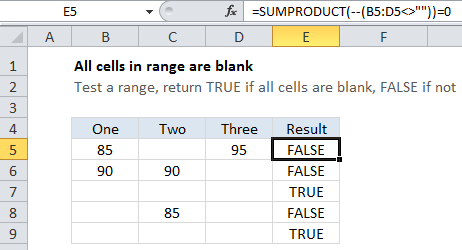# How to return TRUE when all cells in range are blank in Excel

To return TRUE when all cells in a range are blank or empty, you can use a formula based on SUMPRODUCT and a logical test that suits the use case.

## Formula

`=SUMPRODUCT(--(range<>""))=0`## Explanation

In the example shown, the formula in E5 is:

`=SUMPRODUCT(--(B5:D5<>""))=0`

### How this formula works

Working from the inside out, this formula contains an expression inside SUMPRODUCT that tests each cell in a range like so:

`--(B5:D5<>"")`

Inside the parentheses, the result of B5:D5<>”” looks like this:

Also See:   Highlight duplicate rows in Excel

{TRUE,FALSE,TRUE}

The double hyphen then converts the TRUE FALSE values to one’s and zeros:

{1,0,1}

Note that the 1’s in this array correspond to cells that are not blank or empty. Then, with only one array to work with, SUMPRODUCT simply multiples these values together and returns the result.

Any time the result is greater than zero, we know that not every cell in the range is blank. To force the formula to return TRUE if every cell is blank, and FALSE if not, we simply add =0 to the end of the formula.

Also See:   Count day of week between dates in Excel